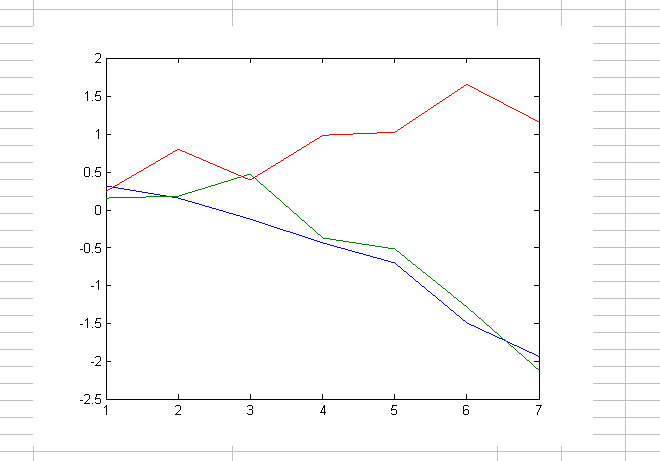## Exchange Bioinformatics Data Between Excel and MATLAB

### Use Excel and MATLAB Together

• Connect Excel with the MATLAB® Workspace to exchange data

• Use MATLAB and Bioinformatics Toolbox™ computational and visualization functions

Note

The Excel file used in the following example contains data from DeRisi, J.L., Iyer, V.R., and Brown, P.O. (Oct. 24, 1997). Exploring the metabolic and genetic control of gene expression on a genomic scale. Science 278(5338), 680–686. PMID: 9381177. The data was filtered using the steps described in Gene Expression Profile Analysis.

### Set System Path and Enable Add-In

3. Close MATLAB and Excel if they are open.

1. Download the provided `Filtered_Yeastdata.xlsm` and open it in Excel.

2. In the Excel software, enable macros. Click the Developer tab, and then select Macro Security from the Code group. If the Developer tab is not displayed on the Excel ribbon, consult Excel Help to display it. If you encounter the "Can't find project or library" error, you might need to update the references in the Visual Basic software. Open Visual Basic by clicking the Developer tab and selecting Visual Basic. Then select Tools > References > SpreadsheetLink. If the MISSING: exclink2007.xlam check box is selected, clear it.

### Run the Example for the Entire Data Set

1. In the provided Excel file, note that columns A through H contain data from DeRisi et al. Also note that cells J5, J6, J7, and J12 contain formulas using Spreadsheet Link functions `MLPutMatrix` and `MLEvalString`.

Tip

To view a cell's formula, select the cell, and then view the formula in the formula bar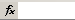at the top of the Excel window.

2. Execute the formulas in cells J5, J6, J7, and J12, by selecting the cell, pressing , and then pressing .

Each of the first three cells contains a formula using the Spreadsheet Link function `MLPutMatrix`, which creates a MATLAB variable from the data in the spreadsheet. Cell J12 contains a formula using the Spreadsheet Link function `MLEvalString`, which runs the Bioinformatics Toolbox `clustergram` function using the three variables as input. For more information on adding formulas using Spreadsheet Link functions, see Create Diagonal Matrix Using Worksheet Cells (Spreadsheet Link).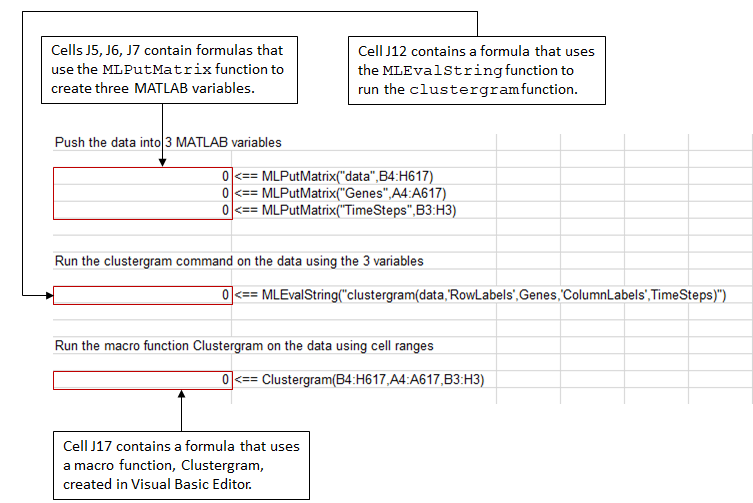3. Note that cell J17 contains a formula using a macro function `Clustergram`, which was created in the Visual Basic® Editor. Running this macro does the same as the formulas in cells J5, J6, J7, and J12. Optionally, view the `Clustergram` macro function by clicking the Developer tab, and then clicking the Visual Basic button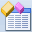. (If the Developer tab is not on the Excel ribbon, consult Excel Help to display it.)

4. Execute the formula in cell J17 to analyze and visualize the data:

1. Select cell J17.

2. Press F2.

3. Press .

The macro function `Clustergram` runs creating three MATLAB variables (`data`, `Genes`, and `TimeSteps`) and displaying a Clustergram window containing dendrograms and a heat map of the data.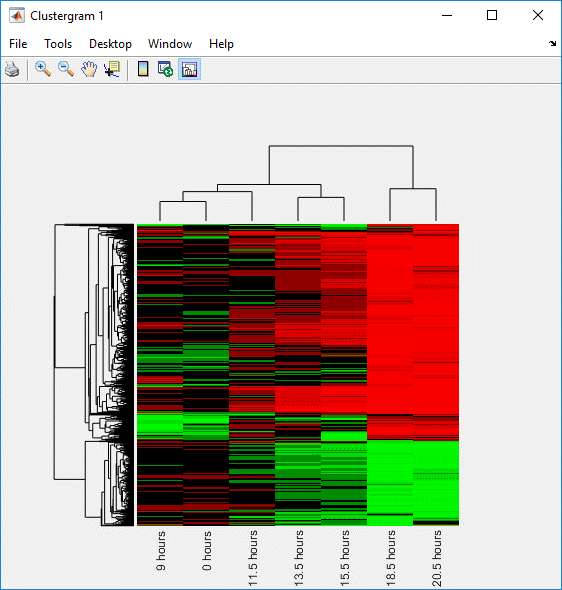### Edit Formulas to Run the Example on a Subset of the Data

1. Edit the formulas in cells J5 and J6 to analyze a subset of the data. Do this by editing the formulas’ cell ranges to include data for only the first 30 genes:

1. Select cell J5, and then press F2 to display the formula for editing. Change H617 to H33, and then press Enter.2. Select cell J6, then press F2 to display the formula for editing. Change A617 to A33, and then press Enter.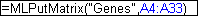2. Run the formulas in cells J5, J6, J7, and J12 to analyze and visualize a subset of the data:

1. Select cell J5, press F2, and then press .

2. Select cell J6, press F2, and then press .

3. Select cell J7, press F2, and then press .

4. Select cell J12, press F2, and then press .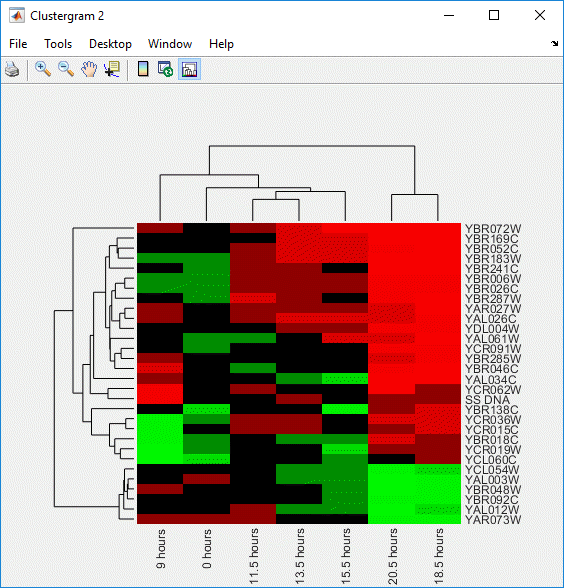### Use the Spreadsheet Link product to Interact With the Data in MATLAB

Use the MATLAB group on the right side of the Home tab to interact with the data:For example, create a variable in MATLAB containing a 3-by-7 matrix of the data, plot the data in a Figure window, and then add the plot to your spreadsheet:

1. Click-drag to select cells B5 through H7.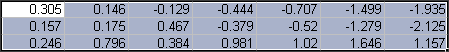2. From the MATLAB group, select Send data to MATLAB.

3. Type YAGenes for the variable name, and then click .

The variable YAGenes is added to the MATLAB Workspace as a 3-by-7 matrix.

4. From the MATLAB group, select Run MATLAB command.

5. Type plot(YAGenes`'`) for the command, and then click .

A Figure window displays a plot of the data.

Note

Make sure you use the `'` (transpose) symbol when plotting the data in this step. You need to transpose the data in `YAGenes` so that it plots as three genes over seven time intervals.

6. Select cell J20, and then click from the MATLAB group, select Get MATLAB figure.